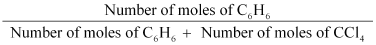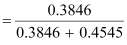# Calculate the mole fraction of benzene in solution containing 30% by mass in carbontetrachloride.

9 years ago

Let the total mass of the solution be 100 g and the mass of benzene be 30 g.
∴Mass of carbon tetrachloride = (100 − 30)g
= 70 g
Molar mass of benzene (C6H6) = (6 × 12 + 6 × 1) g mol−1
= 78 g mol−1

∴Number of moles of C6H6

= 0.3846 mol

Molar mass of carbon tetrachloride (CCl4) = 1 × 12 + 4 × 355
= 154 g mol−1

∴Number of moles of CCl4
= 0.4545 mol
Thus, the mole fraction of C6H6 is given as:= 0.458

APPROVE!

2 years ago
Dear Student,
Please find below the solution to your problem.

Let the total mass of the solution be 100 g and the mass of benzene be 30 g.
∴Mass of carbon tetrachloride = (100 − 30)g
= 70 g
Molar mass of benzene (C6H6)
= (6 × 12 + 6 × 1) g mol−1
= 78 g mol−1
∴Number of moles of C6H6
= 0.3846 mol
Molar mass of carbon tetrachloride (CCl4)
= 1 × 12 + 4 × 355
= 154 g mol−1
∴Number of moles of CCl4
= 0.4545 mol
Thus, the mole fraction of C6H6 is given as:
Number of moles of C6H6 / (Number of moles of C6H6 + Number of moles of CCl4)
= 0.3846 mol / (0.3846 mol + 0.4545 mol)
= 0.458

Thanks and Regards